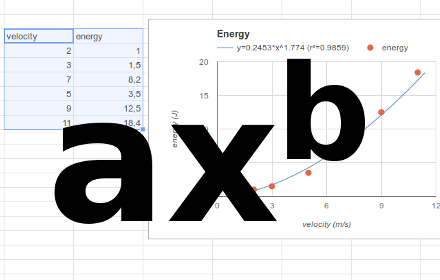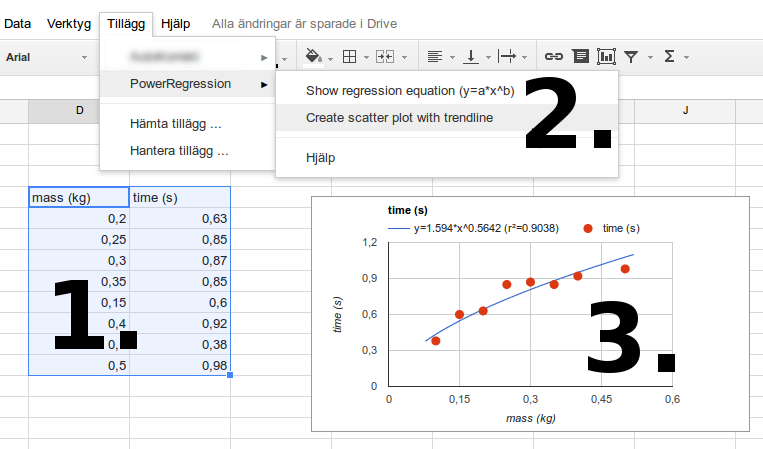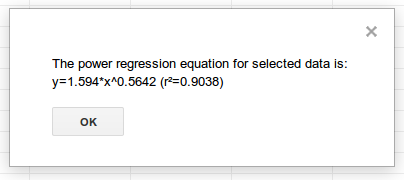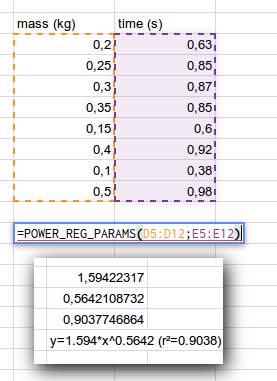During the summer of 2017 Google made an update to their charts. It is now possible to fit a power regression trendline to your data directly from the charts editor. That means there is no longer any need for this add-on. Thanks to everyone who have used it. /Magnus

Calculates and plots a power regression trendline for x- and y-values in a spreadsheet.
With this add-on you will be able to fit a power regression equation, y = a · x
b, to your spreadsheet data and draw its trendline in a scatter plot. The add-on also includes a custom function that puts each of the equation's parameters (a and b) into a cell of your spreadsheet so you can use them for further calculations.

Many correlations follow a mathematical power law, y = a · xb, and with this add-on you can calculate and plot the best fitted power equation to your data.

## Content:

Install

Make a scatter plot with a power regression trendline

Get the equation and r² value for a power regression

Custom function

Nota bene!

Contact

## Make a scatter plot with a power regression trendline

1. Select x- and y-values in two columns from your spreadsheet. The x-values must be in the column just to the left of the column of y-values. Only positive numbers are allowed as x:s and y:s. Cells in the first row may contain captions.

3. Wait a second for the diagram to be created.## Get the equation and r² value for a power regression

1. Select x- and y-values in two columns from your spreadsheet. The x-values must be in the column just to the left of the column for y-values. Only positive numbers are allowed as x:s and y:s. Cells in the first row may contain captions.

3. A dialog window with the equation y=a*x^b and the r² value will be displayed.## Custom function

This add-on also contains a custom function that you can use in your spreadsheet. Only positive numbers are allowed as x:s and y:s.

POWER_REG_PARAMS(x_range, y_range)

This function will return the parameters a and b for the best fitted power equation (y=a*x^b) for the provided x- and y-lists. It also returns the -value and the equation as a whole.## Nota bene!

The diagram will not update when you change values in your original data. You will have to make a new diagram. (I'm sorry, but I have not been able to solve this.)

## Contact

magnus.kson@gmail.com